## Discount Electronic Components

Need assistance? Give us a call. (631) 234-3857

# DUAL POTENTIOMETERS 2 WATT

The most popular style of dual potentiometer has a single shaft to control both elements of the potentiometer. This has been the industry standard for many years. This style of pot is available in various tapers however the 3 most popular are:

• Linear Taper
• Audio Taper (modified logarithmic)
• Reverse Logarithmic Taper (counter-clockwise logarithmic)

Standard ohm values for this style of pot start at 50 ohms and go as high as 5 Million (Meg) ohms. They are very durable and have been designed to run from 25,000 cycles up to 100,000 cycles.

They are available in both commercial grade and military grade. Some of the most popular prefixes for the commercial grade 2 watt dual pots are CCU, JJU, KKU and JD1N. Military grade 2 watt dual pots start with the prefix 2RV7.

The most popular size bushing is 3/8 (0.375) inches and shaft length is 1 5/8 (1.625) inches for an overall length of 2 inches. Standard shaft diameter is 1/4 (0.25) inches and the standard overall diameter (OD) is 1 inch.
Modification of the shaft length is something that is often done without any trouble.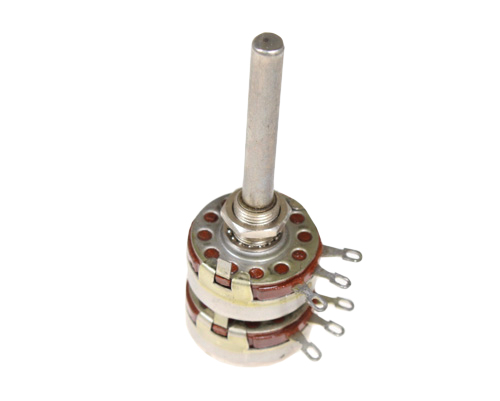CCU series (2 inch long shaft)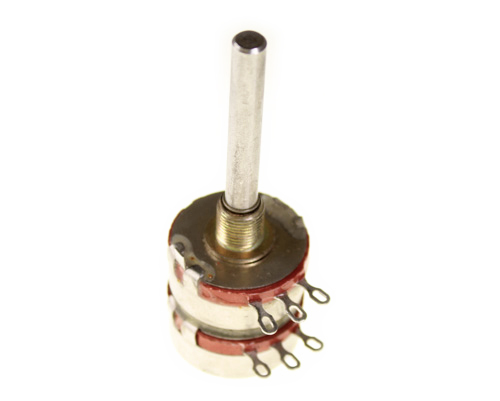KKU series (2 inch long shaft)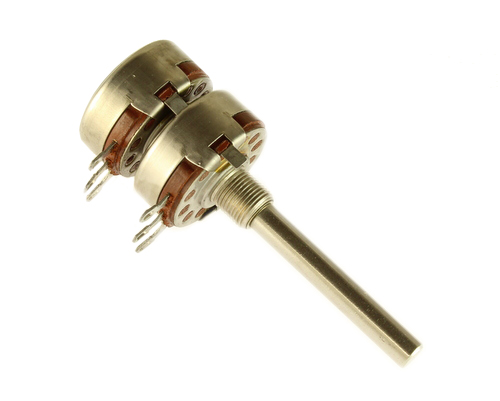JD1N (2 inch long shaft)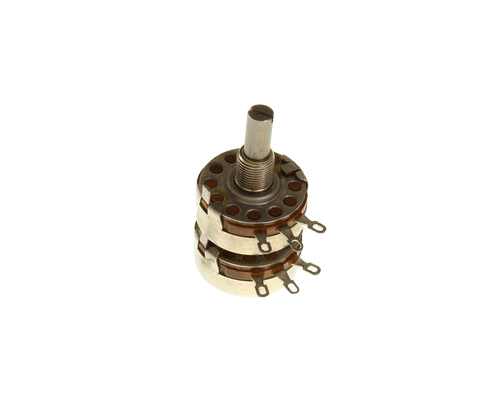JD1N series with 7/8 inch shaft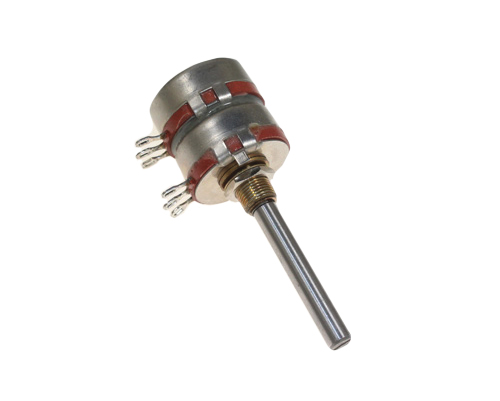2RV7 series with 2 inch shaft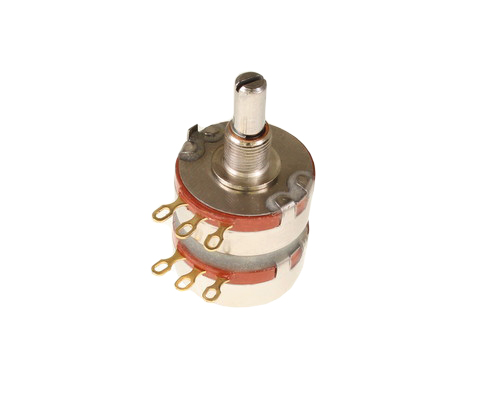2RV7 series with 7/8 inch shaft

## CCU and JJU Series Part Number Breakdown

 CCU2531 / JJU2531 CCU 253 1 | | | Series Resistance Tolerance

Series Name:

• CCU / JJU Style Dual Potentiometer

Resistance:

• 25 = first 2 numbers are significant digits
• 3 = number of zeroes

Tolerance:

• 1 = 10%
• 2 = 20%

## KKU Series Part Number Breakdown

 KKU2521S64 KK U 252 1 S 64 | | | | | | | | Series Bushing Switch Taper Resistance Tolerance Shaft Style Shaft Length

Series Name:

• KKU Style Dual Potentiometer

Bushing:

• blank = Standard
• L = Locking
• W = Panel and shaft seal

Switch:

• blank = None
• S = SPST switch

Taper:

• U = linear
• A = logarithmic
• B = rev. logarithmic

Resistance:

• 25 = first 2 numbers are significant digits
• 2 = 3rd number is the number of zeros

Tolerance:

• 1 = 10%
• 2 = 20%

Shaft Style:

• S = Slotted
• F = Flatted
• R = Round

Shaft Length:

• 16 = 1/2 in
• 20 = 5/8 in
• 24 = 3/4 in
• 28 = 7/8 in
• 32 = 1 in
• 40 = 1 1/4 in
• 48 = 1 1/2 in
• 64 = 2 in
• 80 = 2 1/2 in
• 96 = 3 in

## JD1N Series Part Number Breakdown

 JD1N056S105UA JD 1 N 056 S 105 U A | | | | | | | | Series Locating Lug Option Bushing Shaft Length Shaft Style Resistance Linearity Mounting Hardware

Series Name:

• JD Style Dual Potentiometer

Locating Lug Option:

• 1
• 2
• 3
• 4

Bushing:

• R = plain 0.125 in long
• G = plain 0.25 in long
• N = plain 0.375 in long
• H = plain 0.5 in long
• L = standard locking 0.5 in long
• M = special locking 0.375 in long
• K = special locking 0.5 in long
• F = shaft watertight 0.25 in long
• D = shaft watertight 0.375 in long
• W = shaft watertight 0.5 in long
• P = panel watertight 0.281 in long
• E = panel watertight 0.406 in long

Shaft Length:

• 032 = 1/2 in.
• 056 = 7/8 in.
• 200 = 2.0 in.

Shaft Style:

• S = Slotted
• F = Flatted
• P = Round

Resistance:

• 10 = first 2 numbers are significant digits
• 5 = 3rd number is the number of zeros

Linearity:

• U = Linear 10%
• M = Linear 20%
• A = Log 10%
• R = Log 20%
• B = Rev. Log 10%
• T = Rev. Log 20%

Mounting Hardware:

## 2RV7 Series Part Number Breakdown

 2RV7NYSD103103A 2RV7 N Y S D 103103 A | | | | | | | Series Bushing Temperature andMoisture Characteristics Shaft Style Shaft Length Resistance Linearity andTolerance

Series Name:

• 2RV7 MIL Style Dual Potentiometer

Bushing:

• N = standard
• L = locking
• S = panel & shaft seal

Temperature and Moisture Characteristics:

• Y = as per MIL-R-94

Shaft Style:

• S = Slotted
• F = Flatted

Shaft Length:

• B = 1/2 inch
• A = 5/8 inch
• D = 7/8 inch
• G = 1 1/4 inch
• J = 2 inch
• K = 2 1/2 inch

Resistance:

• 10 = first 2 numbers are significant digits
• 3 = 3rd number is the number of zeros

Linearity:

• A = Linear 10%
• B = Linear 20%
• C = Log 10%
• D = Log 20%
• E = Rev. Log 10%
• F = Rev. Log 20%